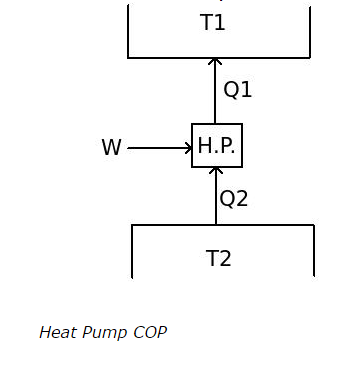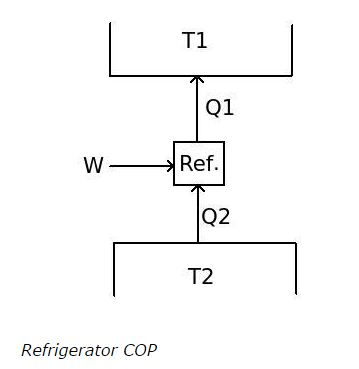# Refrigeration - MCQs with Answers

## Refrigeration - MCQs with Answers

Q1. The heat pump

a. receives heat from high temperature region and discharge it to low temperature region with production of useful work

b. receives heat from low temperature region and discharge it to high temperature region with production of useful work

c. receives heat from high temperature region and discharge it to low temperature region with utilization of external work

d. receives heat from low temperature region and discharge it to high temperature region with utilization of external work

ANSWER: d. receives heat from low temperature region and discharge it to high temperature region with utilization of external work

Q2. What is correct formula for calculating COP of heat pumpa. [COP]H. P. = Q1 / W
b. [COP]H. P. = Q2 / W
c. [COP]H. P. = W / Q1
d. [COP]H. P. = W / Q2

ANSWER: a. [COP]H. P. = Q1 / W

Q3. How is the COP of a refrigerator calculated?a. [COP]Ref. = Q1 / W
b. [COP]Ref. = Q2 / W
c. [COP]Ref. = W / Q1
d. [COP]Ref. = W / Q2

ANSWER: b. [COP]Ref. = Q2 / W

Q4. Where is main concentration in refrigeration for its calculation?

a. high temperature reservoir
b. low temperature reservoir
c. both high temperature and low temperature reservoirs
d. none of the above

Q5. What is the relation between [COP]H. P. and [COP]Ref.?

a. [COP]H. P. = [COP]Ref.
b. [COP]H. P. + [COP]Ref. = 1
c. [COP]H. P. – [COP]Ref. = 1
d. [COP]H. P. × [COP]Ref. = 1

ANSWER: c. [COP]H. P. – [COP]Ref. = 1

Q6. What is the effect of decrease in temperature difference between two reservoirs of heat pump on its COP?

a. COP increases with decreases in temperature difference between two reservoirs
b. COP decreases with decreases in temperature difference between two reservoirs
c. COP does not affected by change in temperature difference between two reservoirs
d. cannot say

ANSWER: a. COP increases with decreases in temperature difference between two reservoirs

Q7. The ideal refrigeration cycle is like

a. Carnot cycle
b. reversed Carnot cycle
c. Rankine cycle
d. reversed Rankine cycle